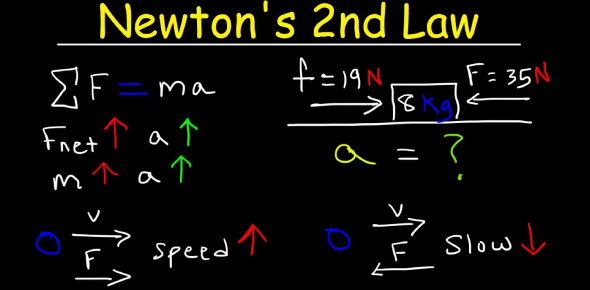# Do You Know Newton's Second Law Of Motion? Trivia Quiz

10 Questions | Attempts: 1214SettingsNewton's motion laws are three physical laws that describe the relationship between the motion of the body and the forces acting on it. The second directive asserts that the rate of the momentum of a body over time is directly proportional to the force applied. If you want to learn more about Newtons' second law of motion, complete this quiz.

• 1.
Newton's second law of motion states that when a net force acts on an object the object will __________ in the direction of the force.
• A.

Roll

• B.

Push back

• C.

Accelerate

• D.

Terminal velocity

• 2.
What is the formula for Newton's second law of motion?
• A.

F=ma

• B.

F=mph

• C.

F=d/t

• D.

F=ml

• 3.
How much force is needed to accelerate a 200 kg motorcycle at 4 m/s2?
• A.

600 N

• B.

800 N

• C.

10000 N

• D.

12000 N

• 4.
If a 70 kg skydiver jumped out of a plane, what would be the force of his falling?
• A.

545 N

• B.

7.14 N

• C.

0.14 N

• D.

686 N

• 5.
Gravity on Earth causes falling objects to accelerate at a rate of 9.8 m/s2.
• A.

True

• B.

False

• 6.
Terminal Velocity is the point at which a free-falling object cannot accelerate any more due to what two are forces working against each other?
• A.

Gravity and Air Resistance

• B.

Gravity and Mass

• C.

Air Resistance and Mass

• D.

Gravity and Newtons

• 7.
Newton's second law of motion means that a basketball would require more force to accelerate than a bowling ball.
• A.

True

• B.

False

• 8.
If 20 N of force were applied to a 16 kg bowling ball, at what rate would it accelerate?
• A.

1.25 m/s2

• B.

0.8 m/s2

• C.

320 m/s2

• D.

45 m/s2

• 9.
If a ping pong ball falls off a table and hits the ground with 4 N of force applied to it, what is its mass?
• A.

2.45 kg

• B.

0.41 kg

• C.

39.2 kg

• D.

4.3 kg

• 10.
Increasing the mass but keeping the force constant causes the acceleration to decrease.
• A.

True

• B.

False

## Related TopicsBack to top
×

Wait!
Here's an interesting quiz for you.